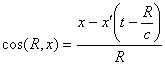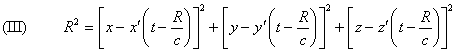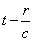### Home | Up One Level | Previous | Next

WALTER RITZ

#### Annales 217 (Oeuvres 377)

We saw that Lorentz’s theory is expressed, in final analysis, by the statement of an elementary law of action between two charged elements of volume. A mobile corpuscle P’(x’,y’,z’), carrying the charge e’, exerts on another P of charge e placed in xyz a force depending on the speed v of this latter, of  the direction, . . . and of the radius R of the wave emitted by P’ at the moment t’= t - R/c, and reaching P at instant t; and finally, of the speed v’ and of the acceleration w’ of P’ in t’. We have (the system of coordinates being at rest in relation to the ether),

the equation which defines R as an implicit function of x, y, z, t when the functions x’=x’(t’), y’,z’ are given. We then conclude### The equations of motion we just wrote are of the second order like those of mechanics, but because of the argument, they are, at the same time, functional equations and this very complicated, mixed form cannot be definitive.

 Göttinger Nachrichten, 1903, p. 132 and following. The formula includes some restrictions without importance for our purposes.

 H. von Helmholtz, Wissenschaftliche Abhandlungen, vol. I, Leipzig, 1882, pp. 553, 636, 656.

 See the formula (XVII) by Schwarzschild in the first Part.